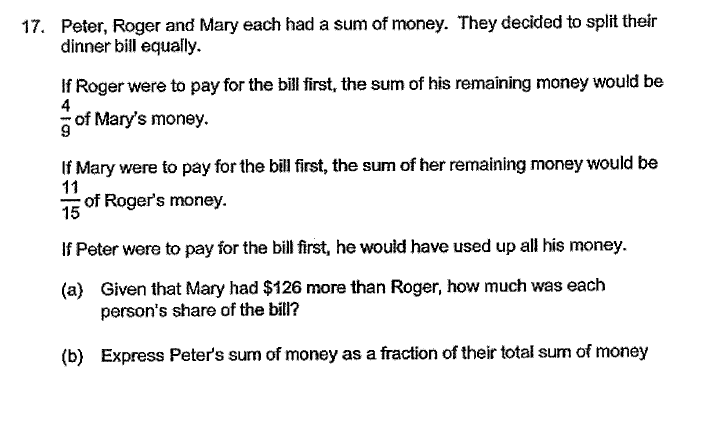# QuestionSource: 2020 prelim

Roger’s Remainder : Mary’s Original : Total

4                :           9                  :    13

Roger’s Original : Mary’s Remainder : Total

15           :           11                     :    26

Roger’s Remainder + Mary’s Original  = Roger’s Original + Mary’s Remainder. The reason is because it does not matter whether Roger paid for the bill or Mary paid for the bill, the total of Roger’s and Mary’s  must be less by the bill amount.  To help you better understand, I am going to use some fictitious values.

Lets say Roger had \$150 and Mary had  \$180 at first and the bill cost \$30. If Roger paid for the bill, Roger will have \$120 left whereas Mary will have \$180. The total in the end will be \$300. If Mary paid for the bill, Roger will have \$150 whereas Mary will have \$150. The total in the end is still \$300

As you can see, the total of Roger and Mary in the end is still the same. Since the total must be equal, we must make the total for both ratios the same by multiplying the top ratio by 2.

Roger’s Remainder : Mary’s Original : Total

8                :           18                  :    26

Roger’s Original : Mary’s Remainder : Total

15           :           11                     :    26

Since Roger’s original money is 15 units and Mary’s original money is 18 units, we can conclude that \$126 is 3 units and 1 unit is \$42.

If you observe the ratio carefully, you will realised that the total cost of the bill is 7 units. (15 units – 8 units or 18 units – 11 units). Therefore, the total cost of the bill is \$294 (7 x \$42)

Hence, the amount each person supposed to pay is \$98 (\$294/3)

a) Ans : \$98

Since Peter will use up all his money when he pay for the bill, we can conclude that Peter’s money is \$294

Roger’s money is \$630 (42 x 15)

Mary’s money is \$756 (18 * \$42)

Total of all 3 people is \$1680

294/1680 = 7/40

b) Ans : 7/40